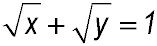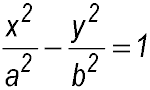Dr. J's Maths.com
Where the techniques of Maths
are explained in simple terms.

Calculus - Differentiation - Implicit Differentiation.
Test Yourself 1.

 Direct differentiation. Use implicit differentiation to findDerive the equation of the following hyperbolaFind the derivative of y2 = x2y + 1. Stationary points. Find the coordinates of any stationary points for the curve y3 + 2xy + x2 + 2 = 0. Find the coordinates of the points where the tangent to the curve x2 + 2xy + 3y2 = 8 is horizontal. Gradients. Find the equation of the tangent to the curve defined by x2 - xy + y3 = 5 at the point (2, -1). Find the coordinates of the point where the tangent to the curve x2 - y2 + xy + 5 = 0 is parallel to the line y = x. A plane curve is defined implicitly by the equation x2 + 2xy + y5 = 4. This curve has a horizontal tangent at P (x, y). Show that x is a root of the equation x5 + x2 + 4 = 0.